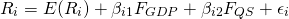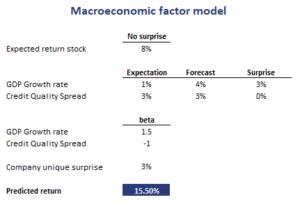# Macroeconomic Factor Models

Macroeconomic factor models are a kind of risk models used to model asset returns and risk. To do this, macroeconomic models make use of macroeconomic risk factors such as GDP, interest rates, inflation, and other factors. Rather than using the macro data itself, multifactor models use the factor surprise. The factor surprise is defined as the difference between the realized value of the factor and its consensus predicted value.

On this page, we discuss the an example of a macroeconomic factor model formula and illustrate how to use a macroeconomic factor model once the parameters have been estimated.

## Macroeconomic factor formula

There are many different macroeconomic factor models available. The formulas for each factor model will be different. To illustrate what a model may look like, let’s consider a simple two-factor model. In this factor model, assets’ returns are explained by surprises in GDP growth rates (GDP) and credit quality spreads (QS). The macroeconomic factor formula in that case equalswhere Ri is the return for asset i (in the absence of any surprises), FGDP and FQS are the factor surprises, the betas (b) are the sensitivities of the stocks to the factors, and epsilon is the firm-specific surprise.

There are a number of points that we have to keep in mind. First, each F is a surprise. It is the difference between the market expectation and the actual value. Thus, if the market expected GDP to increase by 2% and the actual growth was 3%, than the surprise is 1%. Second, each b measures the stock’s sensitivity to the surprise. Third, the epsilon captures the part of the return that cannot be explained by the model.

## Macroeconomic factor model interpretation example

Let’s consider an example. Let’s assume we have we have the same model as above. We have estimated the model using historical data and OLS. The results are reported in the following table. Now, let’s predict the stock’s return if we observe surprises for GDP and the credit quality spread. The results are reported in the table as well.## Summary

We discussed the use of macroeconomic factor model to predict assets’ returns. If we have forecasts for the macroeconomic variables, we can predict the returns of securities.

### Example

Want to have an example in Excel? Download the Excel file: Macroeconomic model